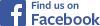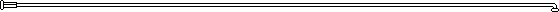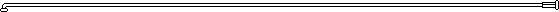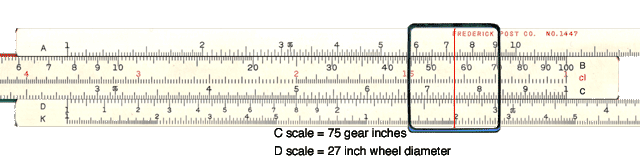Slide Rules and Bicycle GearsTweet Follow @sheldonbrowncomAnyone who can't use a slide rule is a cultural illiterate,
and should not be allowed to vote.
--Robert A. Heinlein## Gear Calculation

Computers are wonderful things, and among their many uses is the rapid calculation of bicycle gearing. I have written (with some volunteer programming help) a Web based system for calculating the gears of multiple speed bicycles. Click here to see it. This and similar systems are excellent for generating charts of multiple gear systems. They are less than ideal for the fixed-gear or singlespeed rider, however. For calculation of single gears, I usually fall back on much older technology, my trusty slide rule!

The slide rule was invented back in 1625 by William Oughtred, based on John Napier's 1614 discovery of logarithms. This was the indespensible tool of mathematicians, scientists, engineers and mathematically literate lay people up until the early 1970s when solid state electronic calculators became available.

The slide rule generally only offers 2-3 digits of precision, but for bicycle gearing that's quite sufficient, and the extra digits provided by many computer applications only obscure things.

Slide rules don't tell you where to put the decimal point. For that you need to apply common sense. The slide rule will give you the significant digits.

Most folks know that a slide rule can be used for multiplication and division, but a less familiar operation is actually more useful for bicycle gear calculations, the "proportion" operation.

The proportion function is particularly useful if you know what gear you want, but aren't sure what combinations of sprockets will give it to you. Using proportions, the slide rule can show you all of the sprocket combinations that provide a given gear value, all at once in a single operation. The image below illustrates how this is done. Note that the only scales of interest here are the "C" scale on the bottom edge of the slider, and the "D" scale just below it on the body of the rule.

The key to understanding proportions is to realize that once you have set the slider to a given position, the ratio between any number on the "C" scale and the corresponding number on the "D" scale is always the same.

The slide rule is set to my favorite fixed gear, 75 inches . It assumes a nominal wheel diameter of 27 inches (you must use the same units top and bottom!) As you can see, the cursor is indicating 75 inches on the "C" scale for the desired gear, and 27 inches on the "D" scale for the actual wheel size. If you think about the origin of gear inches, this ratio of "effective" wheel diameter to actual wheel diameter is going to be the same as the ratio between front and rear sprockets.The image is a "rollover" so if you move your cursor over the left side of the slide rule, you'll see different combintaions highlighted: 36/13, 39/14, 42/15 etc.

This is not limited to gear inches either, although that's the most obvious system. If you move your cursor farther to the right, you'll see that it also displays the same ratio in terms of Gain Ratio (with the Radius Ratio on the "D" scale.) Click here if you're not familiar with the Gain Ratio system.

Move a bit farther to the right, and you'll see the ratio expressed in terms of Meters Development. (Since development is circumference based, the value on the "D" scale is the circumference of the drive wheel, also in meters.)#### Move your mouse cursor over the slide rule image to move the slide rule's cursor, revealing different gear combinations and gear measurement systems that all use the same slider position.## Is this too retro for you?